﻿ 火箭炮闭锁机构撞击失效过程建模及其验证
«上一篇文章快速检索 高级检索

 哈尔滨工程大学学报2018, Vol. 39Issue (9): 1568-1573  DOI: 10.11990/jheu.2017030970

### 引用本文ZHAO Xin, LI Jun, SHEN Xiangjun. Modeling and verification of impact failure in the locking mechanism of a rocket gun[J]. Journal of Harbin Engineering University, 2018, 39(9), 1568-1573. DOI: 10.11990/jheu.201703097.### 文章历史

1. 南京理工大学 机械工程学院, 江苏 南京 210094;
2. 晋西集团技术中心五所, 山西 太原 030000

Modeling and verification of impact failure in the locking mechanism of a rocket gun
ZHAO Xin1, LI Jun1, SHEN Xiangjun2
1. School of Mechanical Engineering, Nanjing University of Science and Technology, Nanjing 210094, China;
2. The Fifth Research Institute of Jinxi Group Technology Center, Taiyuan 030000, China
Abstract: Considering that impact failure of the locking mechanism of a rocket gun occurs in the process of mandatory disengagement, the main factors affecting impact failure were analyzed in combination with general mechanics theory, and the law of this failure process was derived. The corresponding mechanical model was then established. The model was used to analyze the impact failure process of the locking mechanism of a rocket projectile. FE simulation and the established equivalent experiment were combined to verify the law of the impact failure process of the locking mechanism in a real environment. Taken together, the simulation and experimental results agreed with the theoretical analyses. The results showed that the proposed model and method can be applied to theoretical and experimental studies on the impact failure of the locking mechanism and provide a basic method for assessing impact failure performance.
Keywords: rocket gun    locking mechanism    impact failure    mechanical model    drop hammer    equivalent experiment    numerical analysis    performance evaluation

1 闭锁机构撞击失效模型建立 1.1 模型简化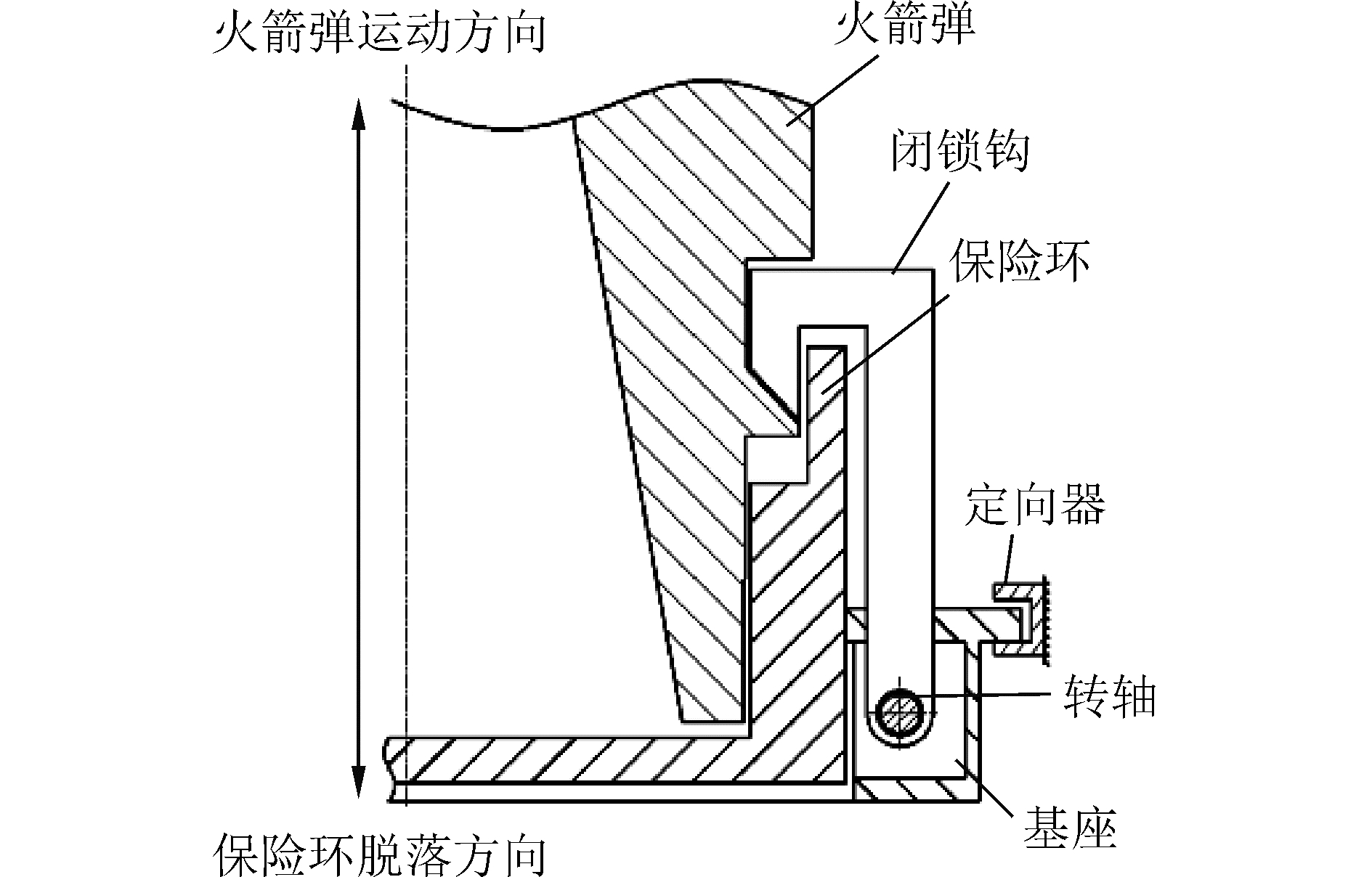Download: 图 1 闭锁机构示意图 Fig. 1 Locking mechanism diagram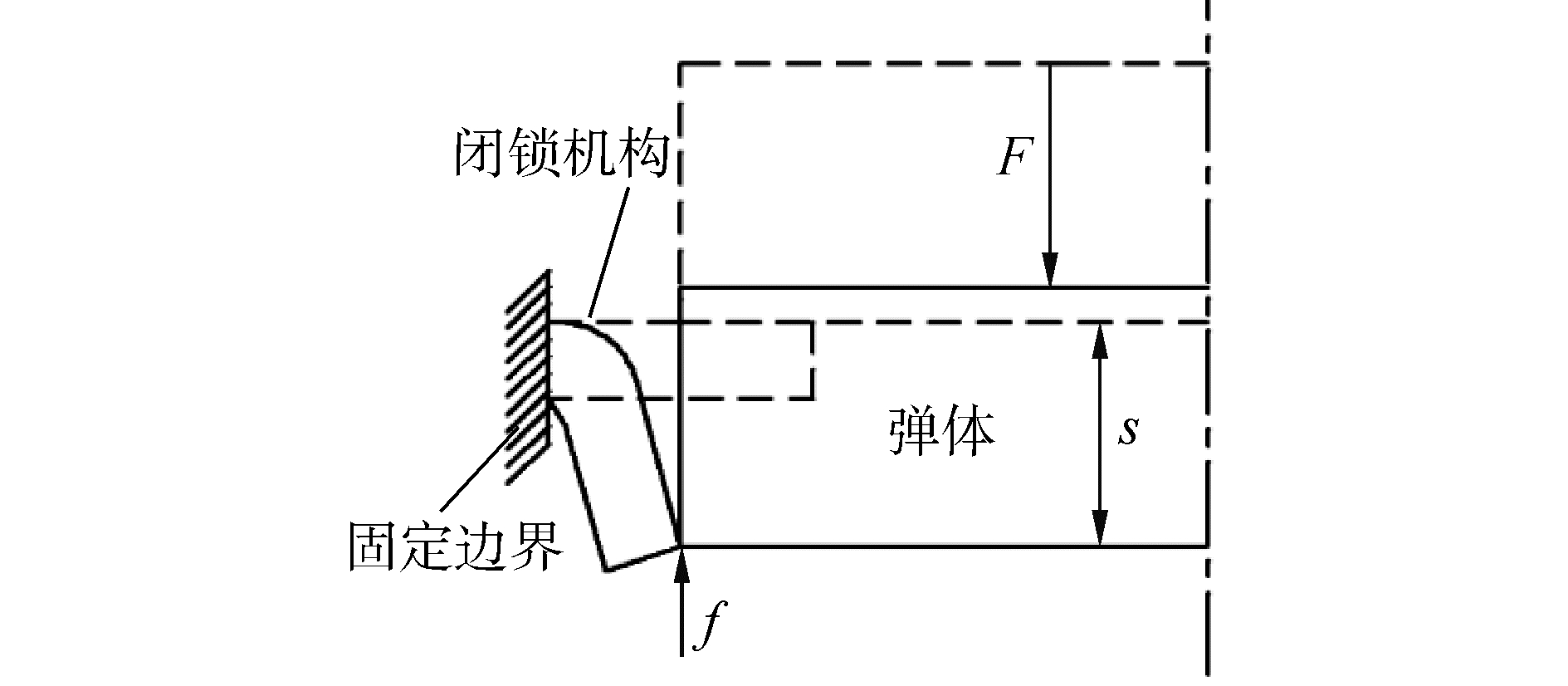Download: 图 2 闭锁机构失效过程原理图 Fig. 2 Locking mechanism failure process schematic diagram
1.2 理论推导

 $\smallint \left( {F - f} \right){\rm{d}}t = M({v_1} - {v_0})$ (1)
 $\smallint F{\rm{d}}s = \frac{1}{2}M(v_1^2 - v_0^2) + \frac{1}{2}{\rm{ }}m{(a{v_1})^2} + U$ (2)
 $\smallint f{\rm{d}}s = \frac{1}{2}m{(a{v_1})^2} + U$ (3)

 $\frac{1}{2}({F_{\rm{c}}} - {f_{\rm{c}}}){t^2} = Ms$ (4)
 ${F_{\rm{c}}}s = \frac{1}{2}Mv_1^2 + \frac{1}{2}m{(a{v_1})^2} + U$ (5)
 ${f_{\rm{c}}}s = \frac{1}{2}m{(a{v_1})^2} + U$ (6)

 $({F_{\rm{c}}} - F{{'}_{\rm{c}}})s = \frac{1}{2}(M + {a^2}m)(v_1^2 - v{'}_1^2)$ (7)
 $({f_{\rm{c}}} - f{{'}_{\rm{c}}})s = \frac{1}{2}{a^2}m(v_1^2 - v{'}_1^2)$ (8)

 ${f_{\rm{c}}} - f{{'}_{\rm{c}}} = \frac{{{a^2}m}}{{M + {a^2}m}}({F_{\rm{c}}} - F{{'}_{\rm{c}}})$ (9)

 ${f_{\rm{c}}} - f{{'}_{\rm{c}}} = 0$ (10)

 $(M + {a^2}m)v_1^2 = (M' + {a^2}m)v{'}_1^2$ (11)
 $({f_{\rm{c}}} - f{{'}_{\rm{c}}})s = \frac{1}{2}(M'v{'}_1^2 - Mv_1^2)$ (12)

a2mMM'时，则

 ${f_{\rm{c}}} - f{{'}_{\rm{c}}} = 0$ (13)

2 有限元验证与等效实验验证 2.1 有限元仿真验证 2.1.1 闭锁机构强制解脱过程有限元模型建立Download: 图 3 闭锁机构作用有限元模型示意图 Fig. 3 Finite element model of locking mechanism
2.1.2 仿真分析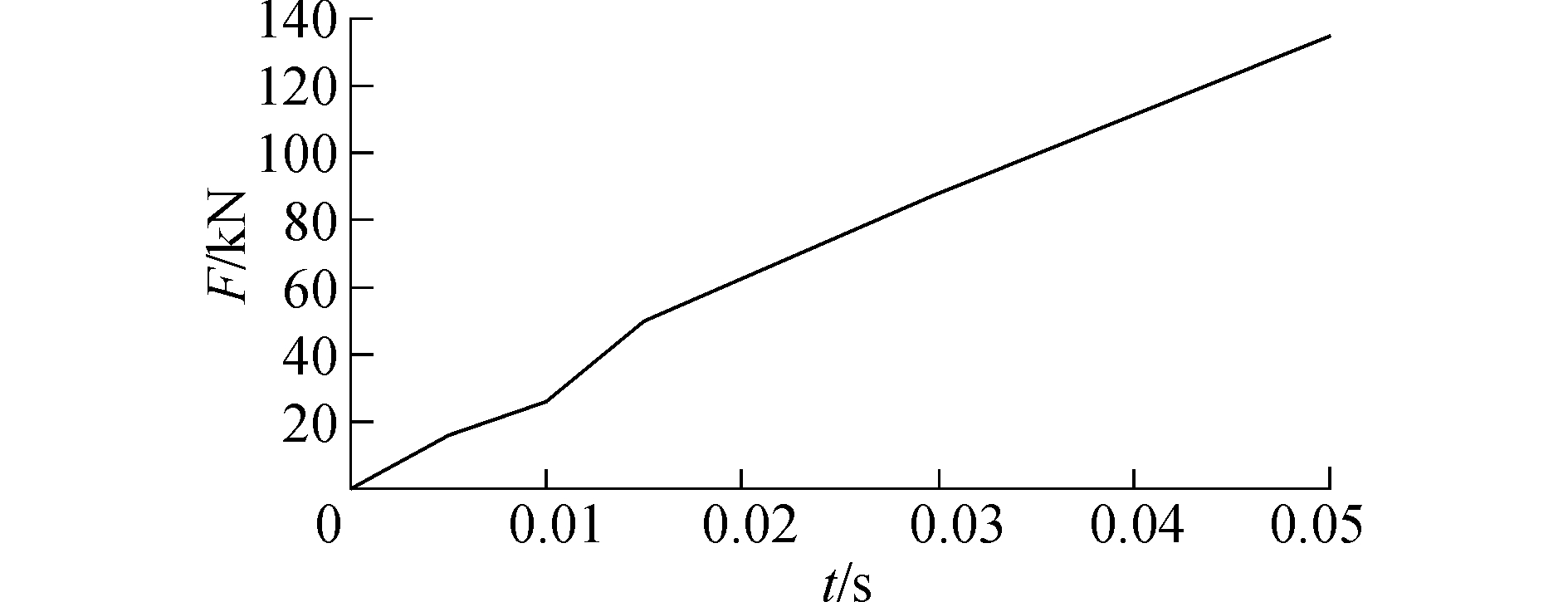Download: 图 4 推力载荷曲线 Fig. 4 Thrust load curve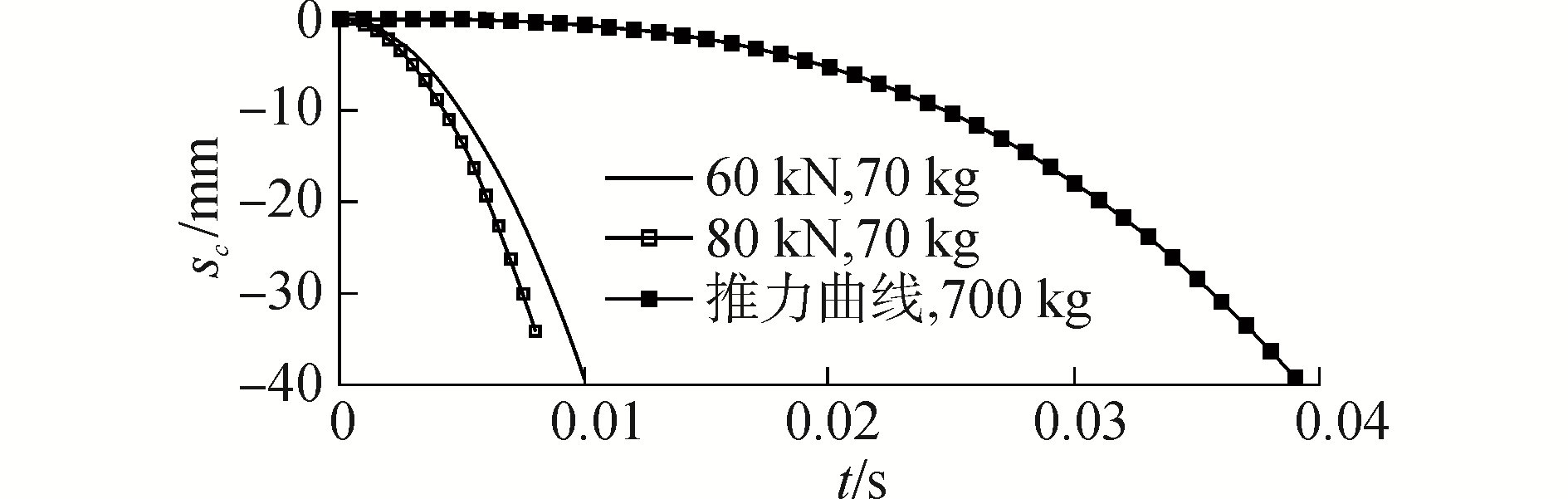Download: 图 5 不同参数下位移-时间仿真曲线 Fig. 5 Displacement-time simulation curves under different parameters表 1 不同参数下的解脱时间 Tab.1 Time of unlock process under different parameters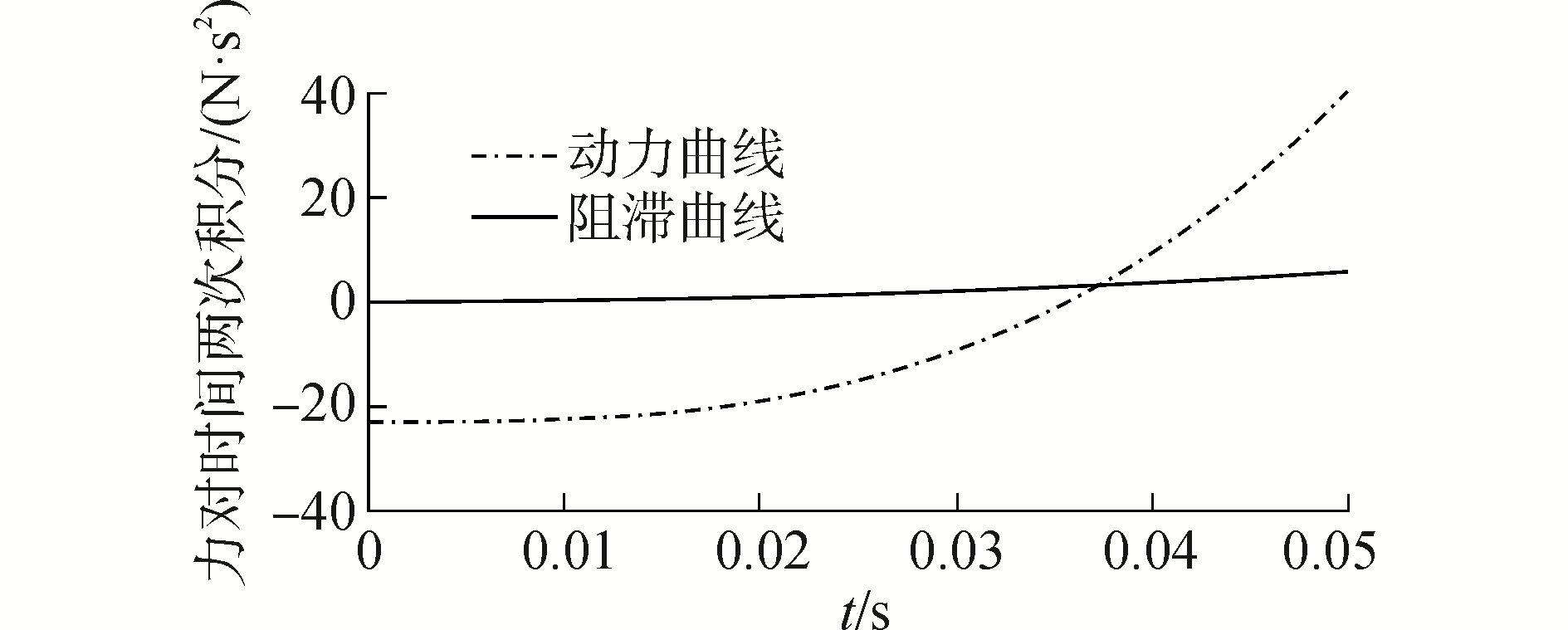Download: 图 6 解脱时间求解曲线 Fig. 6 Time of unlock process solving curve

2.2 等效实验设计及验证

2.2.1 实验设计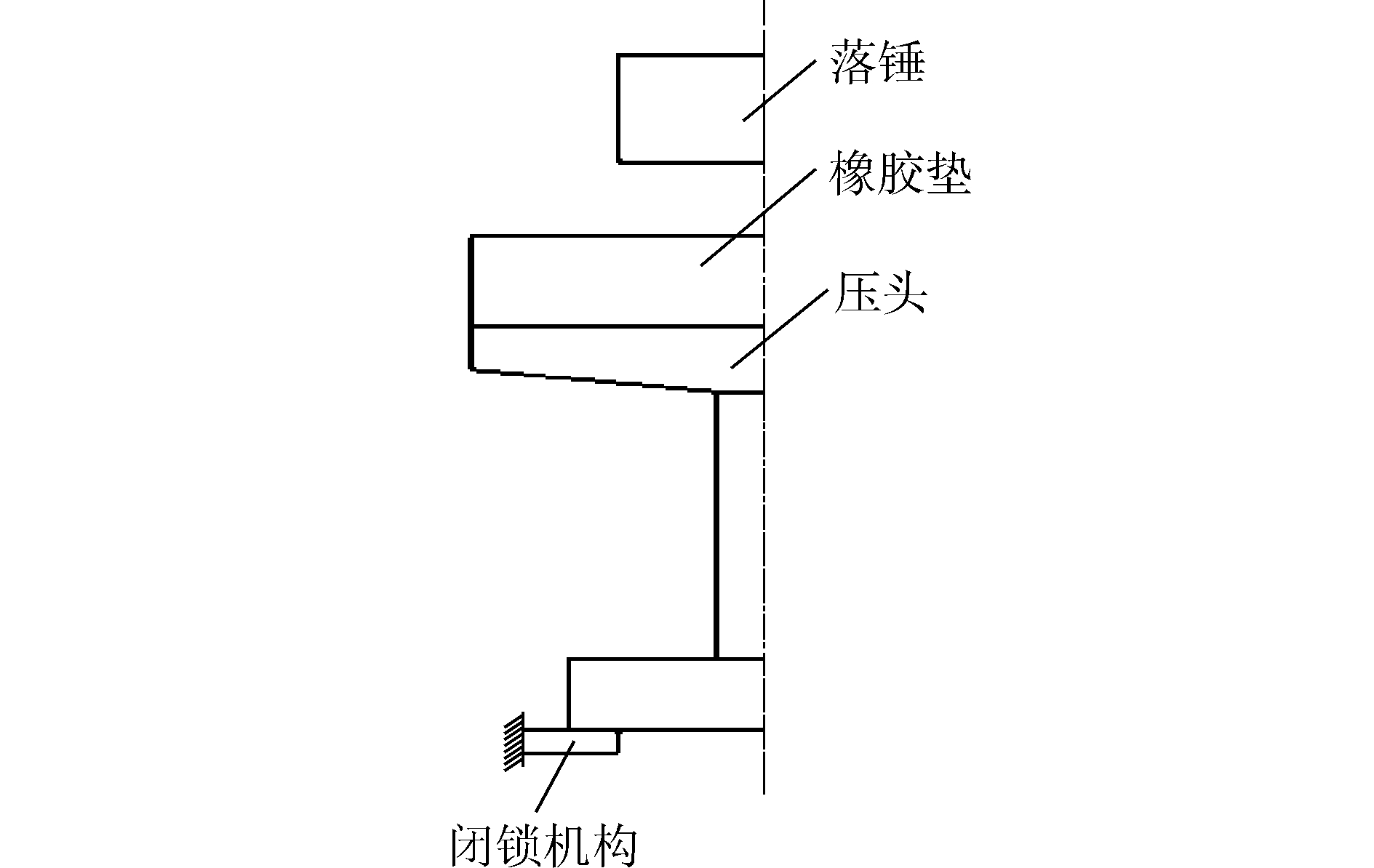Download: 图 7 原理图 Fig. 7 Schematic diagram

1) 落锤：给装置施加冲击载荷；

2) 橡胶垫：起缓冲作用，以延长冲击作用时间；

3) 压头：将载荷传递至闭锁机构。

2.2.2 落锤冲击力作用分析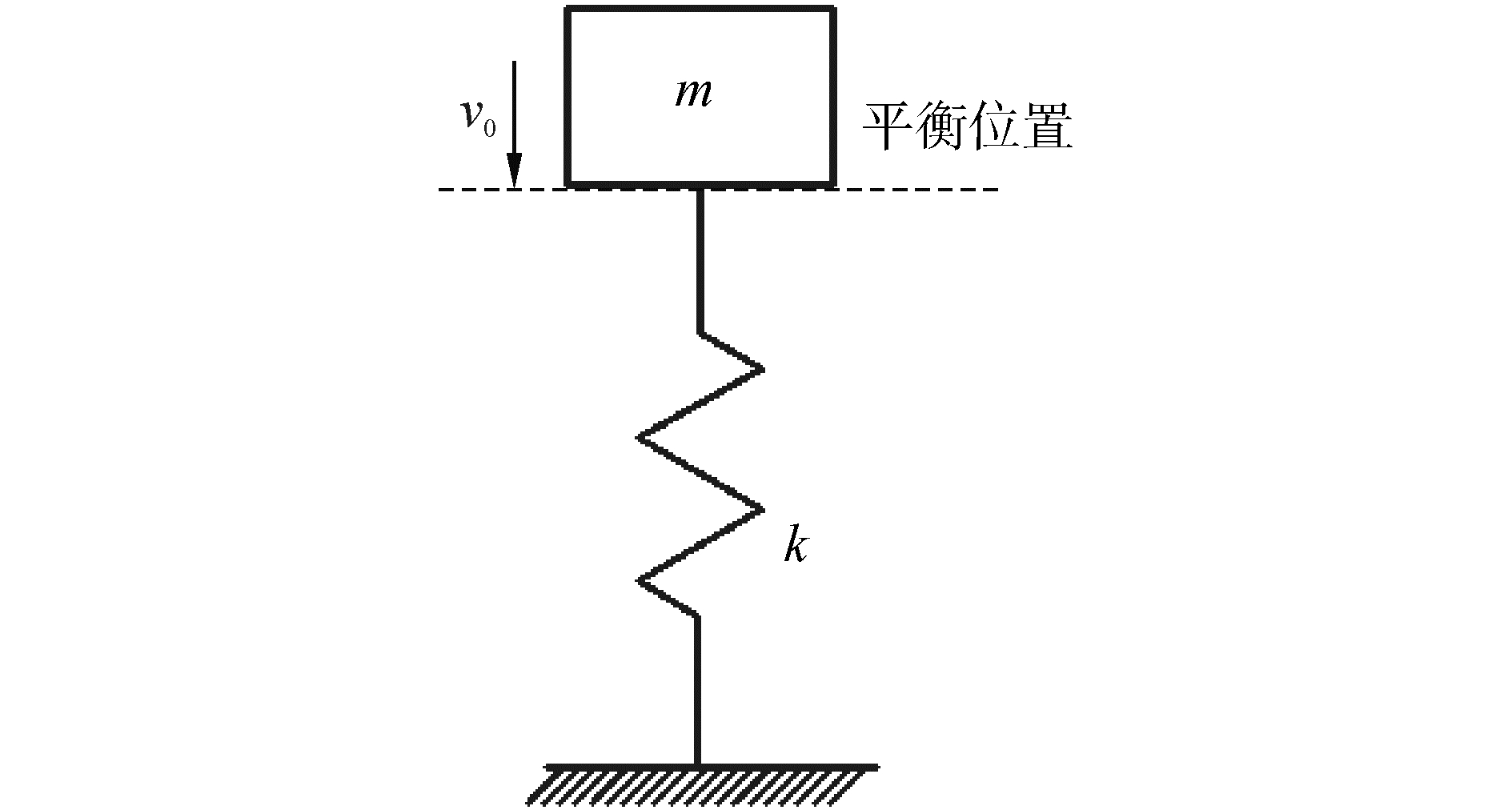Download: 图 8 弹簧振子系统图 Fig. 8 Spring vibration subsystem diagram

 $x\left( t \right) = A\sin ({\omega _{\rm{n}}}t + \varphi )$ (14)
 ${\omega _{\rm{n}}} = \sqrt {k/m}$ (15)
 ${T_{\rm{n}}} = 2{\rm{ \mathsf{ π} }}/{\omega _{\rm{n}}}$ (16)
 $F = kx\left( t \right)$ (17)
 $A = \sqrt {2ghm/k}$ (18)

  (19)

2.2.3 落锤冲击力作用仿真分析验证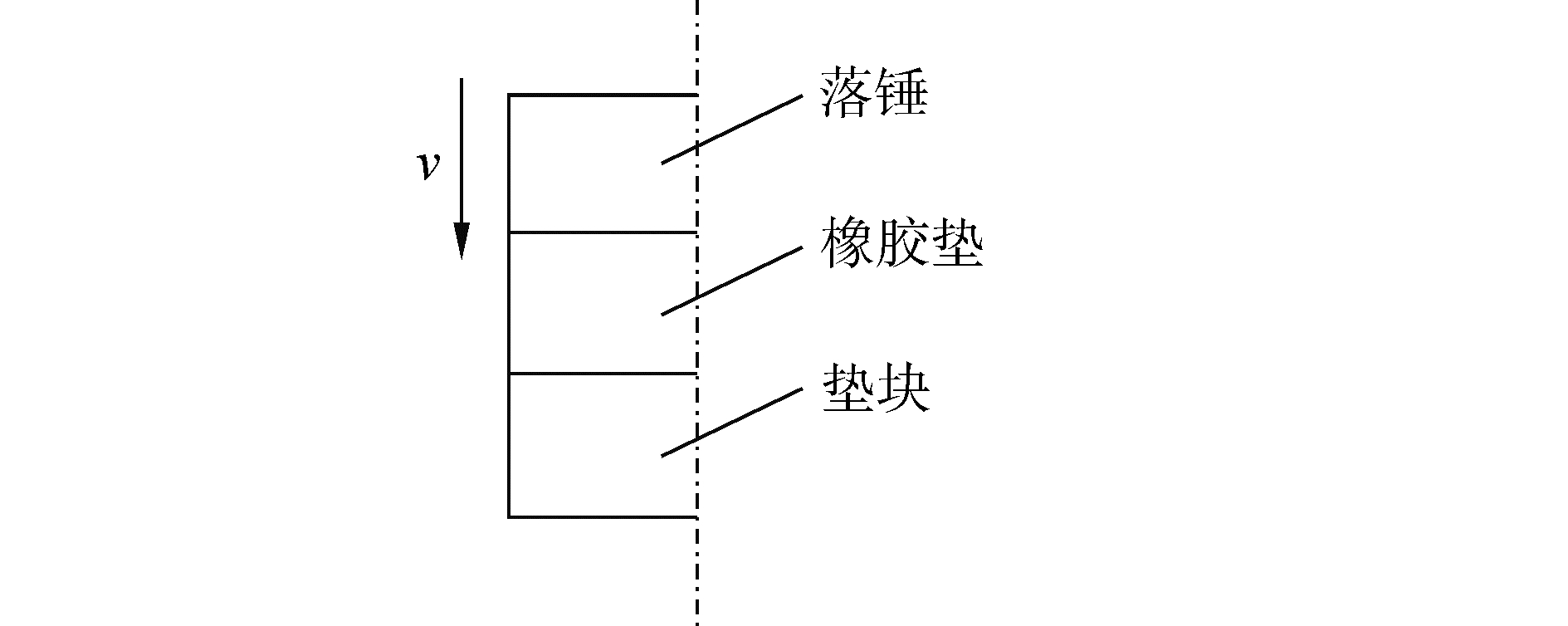Download: 图 9 落锤冲击有限元模型示意图 Fig. 9 Finite element model of drop hammer impact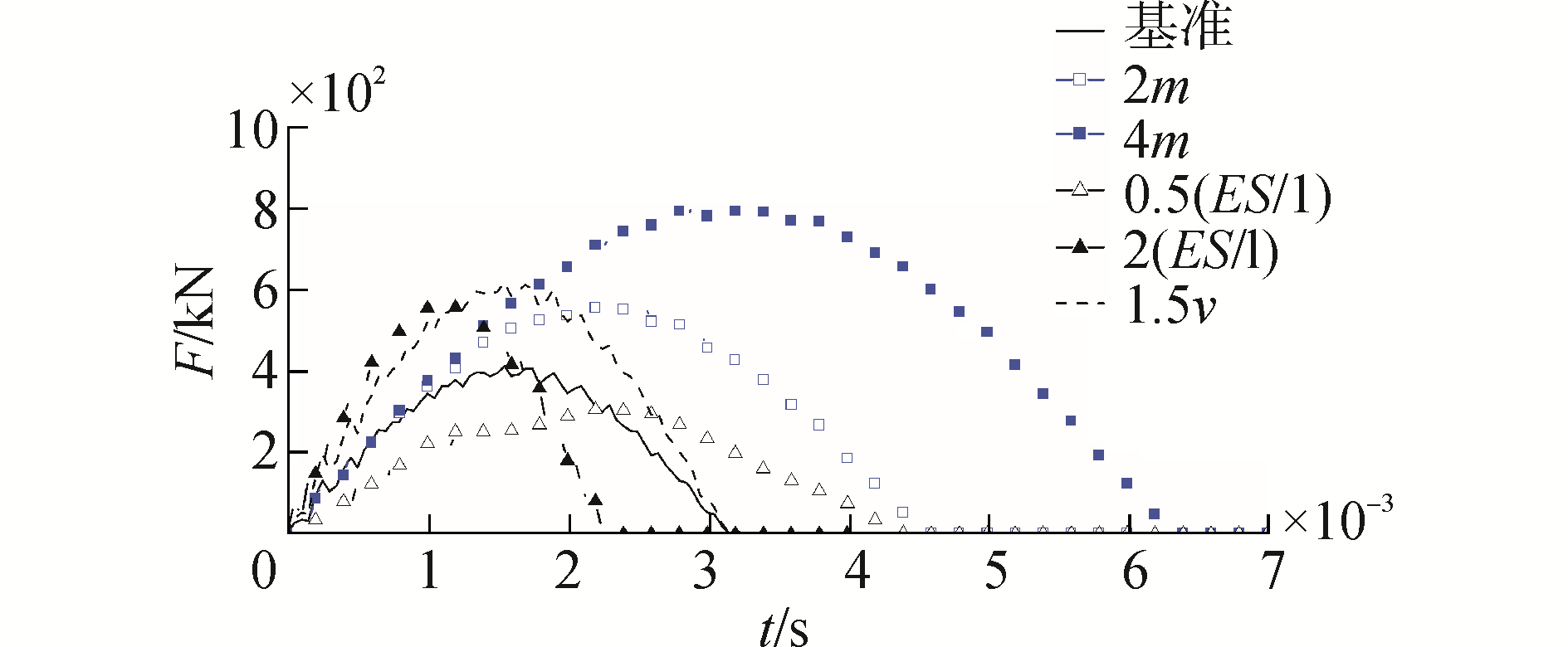Download: 图 10 不同参数下冲击力曲线 Fig. 10 Impact force curves under different parameters表 2 不同参数下冲击力最大值及撞击时间 Tab.2 Maximum impact force and impact time under different parameters in the table

2.2.4 撞击失效过程规律实验验证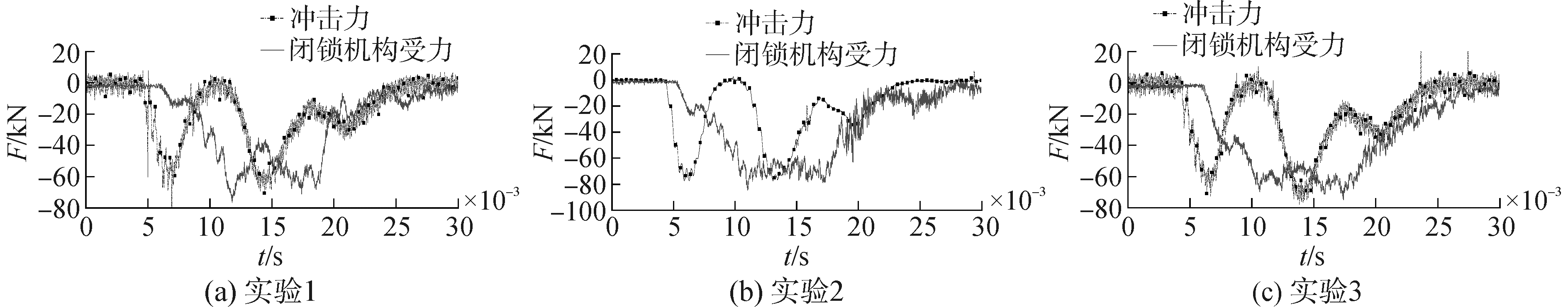Download: 图 11 3次实验冲击力及闭锁机构受力 Fig. 11 Impact force and locking mechanism stress in 3 experiments

3次实验的解脱所用时间由各自闭锁机构受力曲线获得，将冲击力曲线进行两次时间积分，结果如表 3所示。表 3 3次冲击实验结果 Tab.3 Results of 3 impact experiments in the table

3 结论

1) 通过理论推导建立的闭锁机构撞击失效模型及由此获得的撞击失效规律，可为闭锁机构撞击失效过程的理论和实验研究提供基础参考。

2) 当闭锁机构撞击失效过程的应变率不变时，即使冲击载荷和冲击体质量发生变化，闭锁机构提供的平均阻力依然恒定。

3) 依据本文定义的闭锁机构平均阻力，可以评估真实环境条件下火箭弹强制解脱过程中的闭锁机构失效性能，研究结果可初步为闭锁机构的设计及其可靠性和安全性评价提供一种途径。

  李军, 马大为, 曹听荣, 等. 火箭发射系统设计[M]. 北京: 国防工业出版社, 2008: 83-85. (0)  于存贵, 李志刚. 火箭发射系统分析[M]. 北京: 国防工业出版社, 2012. (0)  贺北斗, 林永明, 曹听荣, 等. 火箭发射装置结构分析[M]. 北京: 国防工业出版社, 1997. (0)  万志敏, 王莉, 杜星文. 层合圆柱壳轴向撞击失效行为的试验研究[J]. 哈尔滨工业大学学报, 2001, 33(3): 304-308. WAN Zhimin, WANG Li, DU Xingwen. Failure behavior of laminated composite cylindrical shells under axial impact loading[J]. Journal of Harbin Institute of Technology, 2001, 33(3): 304-308. DOI:10.3321/j.issn:0367-6234.2001.03.009 (0)  王彪, 刘军, 杨帅. 桥墩柔性防撞装置设计及数值分析[J]. 机电工程, 2016, 33(1): 30-36. WANG Biao, LIU Jun, YANG Shuai. Flexible anti-collision device design and numerical analysis on viaduct pier[J]. Journal of mechanical & electrical engineering, 2016, 33(1): 30-36. (0)  张在中, 姚曙光. 城市轨道车辆吸能结构设计[J]. 铁道科学与工程学报, 2013, 10(3): 94-98. ZHANG Zaizhong, YAO Shuguang. Design of energy absorption structure for urban track vehicle[J]. Journal of railway science and engineering, 2013, 10(3): 94-98. DOI:10.3969/j.issn.1672-7029.2013.03.019 (0)  姜华, 王君杰, 贺拴海. 钢筋混凝土梁桥船舶撞击连续倒塌数值模拟[J]. 振动与冲击, 2012, 31(10): 68-73. JIANG Hua, WANG Junjie, HE Shuanhai. Numerical simulation on continuous collapse of reinforced concrete girder bridge subjected to vessel collision[J]. Journal of vibration and shock, 2012, 31(10): 68-73. (0)  崔铁军, 马云东. 超高层建筑受大型高速飞行物撞击所致非连续性坍塌模拟与研究[J]. 计算力学学报, 2016, 33(1): 66-72. CUI Tiejun, MA Yundong. Numerical simulation for the impact of induced discontinuity collapse of high-rise buildings with large high-speed flying object[J]. Chinese journal of computational mechanics, 2016, 33(1): 66-72. (0)  管公顺, 毕强, 张羽. 铝球弹丸高速撞击表面陶瓷化铝板防护结构实验研究[J]. 振动与冲击, 2015, 34(18): 63-68, 93. GUAN Gongshun, BI Qiang, ZHANG Yu. Experimental investigation on aluminum bumper with ceramic surface coating impacted by an aluminum sphere[J]. Journal of vibration and shock, 2015, 34(18): 63-68, 93. (0)  HUANG Jie, MA Zhaoxia, REN Leisheng, et al. A new engineering model of debris cloud produced by hypervelocity impact[J]. International journal of impact engineering, 2013, 56: 32-39. DOI:10.1016/j.ijimpeng.2012.07.003 (0)  GONGSHUN G, BAOJUN P, WEI Z, et al. Crater distribution on the rear wall of AL-Whipple shield by hypervelocity impacts of AL-spheres[J]. International journal of impact engineering, 2008, 35(12): 1541-1546. DOI:10.1016/j.ijimpeng.2008.07.028 (0)  葛庆子, 翁大根, 张瑞甫. 飞机撞击特大型LNG储罐全过程仿真分析[J]. 振动与冲击, 2016, 35(4): 1-7, 21. GE Qingzi, WENG Dagen, ZHANG Ruifu. Whole process simulation analysis of aircraft's crashing into an extra-large LNG storage tank[J]. Journal of vibration and shock, 2016, 35(4): 1-7, 21. (0)  WILT T, CHOWDHURY A, COX P A. Response of reinforced concrete structures to aircraft crash impact[R]. San Antonio, Texas: Southwest Research Institute, 2011. (0)  HUO Jin, HU Zirong, SUN Yuping. Modeling and analysis of concrete-filled steel structure under aircraft impact[C]//ASME 2011 International Design Engineering Technical Conferences and Computers and Information in Engineering Conference. Washington: The American Society of Mechanical Engineers, 2011: 961-965. (0)  陈四春, 李军, 张伟, 等. 火箭炮闭锁机构工作过程瞬态动力学分析[J]. 南京理工大学学报, 2014, 38(5): 608-614. CHEN Sichun, LI Jun, ZHANG Wei, et al. Transient dynamics analysis on working process of rocket lock mechanism[J]. Journal of Nanjing University of Science and Technology, 2014, 38(5): 608-614. DOI:10.3969/j.issn.1005-9830.2014.05.006 (0)  李彦君.发射装置与火箭弹间相互作用非线性动力学分析[D].南京: 南京理工大学, 2012. LI Yanjun. Nonlinear dynamic analysis of the interaction between the launcher and the rocket[D]. Nanjing: Nanjing University of Science and Technology, 2012. http://cdmd.cnki.com.cn/Article/CDMD-10288-1013165674.htm (0)  周彦江, 袁占杰, 刘广生. 某型火箭炮闭锁装置检测研究[J]. 火炮发射与控制学报, 2010(4): 106-109. ZHOU Yanjiang, YUAN Zhanjie, LIU Guangsheng. Study on lock mechanism test in a type of rocket launcher[J]. Journal of gun launch & control, 2010(4): 106-109. DOI:10.3969/j.issn.1673-6524.2010.04.026 (0)  陈国虎, 陈祥龙, 应跃明, 等. 火箭发射装置闭锁力自动检测装置研究[J]. 机电工程, 2015, 32(9): 1210-1213. CHEN Guohu, CHEN Xianglong, YING Yueming, et al. Automatic detecting device for locking force of rocket launcher[J]. Journal of mechanical & electrical engineering, 2015, 32(9): 1210-1213. (0)  张义民. 机械振动[M]. 北京: 清华大学出版社, 2007. ZHANG Yimin. Mechanical vibration[M]. Beijing: Tsinghua University Press, 2007. (0)